# Samacheer Kalvi 6th Maths Solutions Term 3 Chapter 4 Geometry Intext Questions

You can Download Samacheer Kalvi 6th Maths Book Solutions Guide Pdf, Tamilnadu State Board help you to revise the complete Syllabus and score more marks in your examinations.## Tamilnadu Samacheer Kalvi 6th Maths Solutions Term 3 Chapter 4 Geometry Intext Questions

Try this (Text book Page No. 56)

Question 1.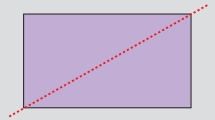The diagonal of a rectangle divides it into two equal halves but it is not a line of symmetry. why?
Solution:
We get two equal halves. But one half of the figure does not coincide exactly with
the other half with the line of symmetry. Therefore it is not a line of symmetry.

Try These (Text book Page No. 57)

Question 1.
Is the dotted line shown in each figure a line of symmetry? If yes put ✓otherwise put ✗ Justify your answer.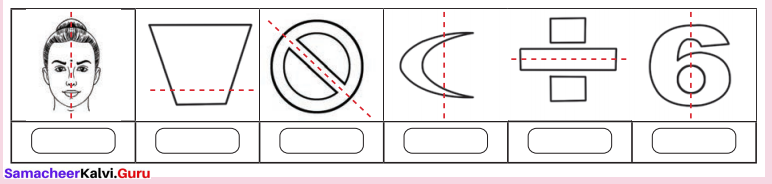Question 2.
Check the following figures for symmetry ? Write YES or NO.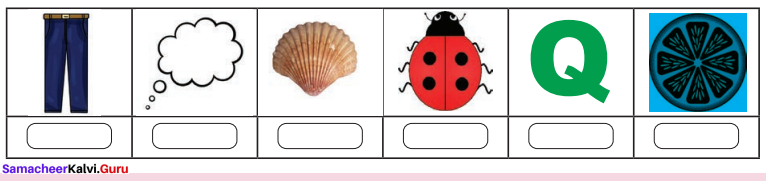Try these (Text Book Page No. 59)

Question 1.
Draw the following figures in a paper. Cut out each of them and fold so that the two parts of each figure exactly coincide.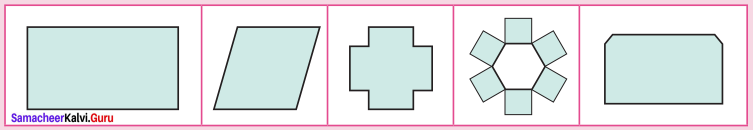i) Which of the above figures have one, two or more lines of symmetry?
ii) Which of the above figures do not have any line of symmetry?
Solution: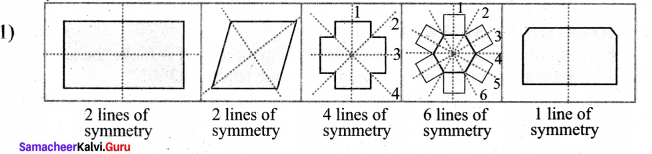2) All have lines of symmetry

Question 2.
Write the numbers from 0 to 9.
(i) Which numbers have a line of symmetry?
(ii) List out the numbers which do not have a line of symmetry.
Solution:
i) Numbers 1 and 3 have one line of symmetry.
ii) Numbers 2, 4, 5, 6, 7 and 9 do not have a line of symmetry7.

Activity (Text Book Page No. 59)

Question 1.
Complete the other half of the following figures such that the dotted line is the line of symmetry.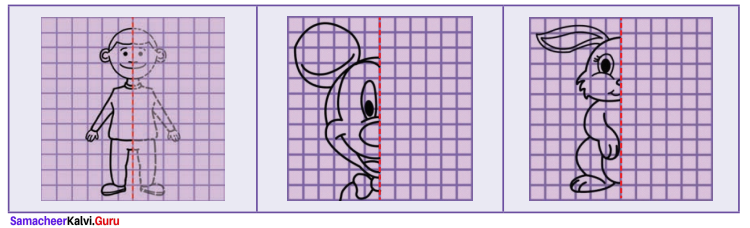Activity to be done by the students themselves

Try this (Text Book Page No. 60)

Question 1.
Which other capital letters of English alphabets look like the same in the mirror?
Solution:
A, H, I, M, O, T, U, V, W, X and Z.Activity (Text book Page No. 62)

Symmetrical figures by ink blots
Step 1 : Take a sheet of paper and fold it into half to make the crease.
Step 2 : Put some ink blots on one side of the crease of the paper.
Step 3 : Fold the paper along the crease and press it.
Step 4 : Open the paper, you will find an imprint of the ink blots on the other part also which is symmetrical about the crease.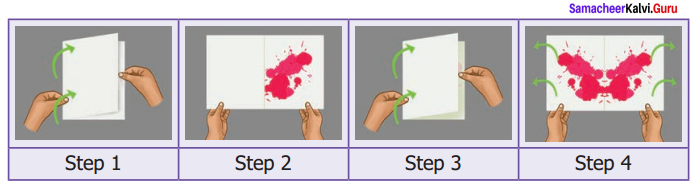Activity to be done by the students themselves

Try These (Text book Page No. 62)

Question 1.
Find the password :
“Kannukkiniyal has a new game app in her laptop protected with a password. She has decided to challenge her friends with this paragraph which contains that password”.
If you follow the steps given below, you will find it.
Steps :
i) Write the above paragraph in capita! letters.
ii) Turn the paper upside down and look at it in the mirror.
iii) The word which remains unchanged in the mirror is the password.
Solution:
i) KANNUKK IN IYAL HAS ANEW GAME APP IN HER LAPTOP PROTECTED WITH A PASSWORD SHE HAS DECIDED TO CHALLENGE HER FRIENDS WITH THIS PARAGRAPH WHICH CONTAINS THAT PASSWORD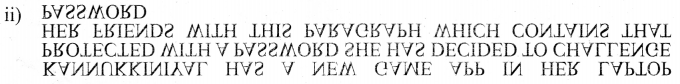iii) “DECIDED” is the password.

Question 2.
Form words using the Setters B, C, D, E,H, I, K, O and X. Write those words in paper in capital letters. Turn it upside down and look at them in the mirror.
Solution:
i) List the letters which have horizontal and vertical line of symmetry
ii) Do the words HIKE, DICE, COOK remain unchanged in the mirror?
iii) The words which you have found that remain unchanged in the mirror are ______, ______, ______
Solution:
B, C, D, E, H, I, K, O, X
i) Vertical lines of symmetry A, H, I, M, O, T, U, V, W, X, Y
Horizontal lines of symmetry B, C, D, E, H, I, K, O, X
ii) Yes
iii) HOOK, KICK, ICETry this (Text book Page No. 64)

Question 1.
Can you identify the object which does not have rotational symmetry in the above situations? Why?
Solution:
1) Computer mouse
2) Trapezium
3) Chair
4) School bags etc.

Question 2.
Suppose, the man hole cover of the water sump is in circular shape
i) The number of ways to dose that circular lid is ___
ii) What is its order of rotational symmetry?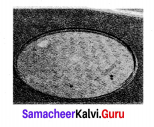Solution:
i) many
ii) Infinite

Activity (Text book Page No. 64)

Question 1.
Find the order of rotational symmetry by fixing the relevant shape in different ways.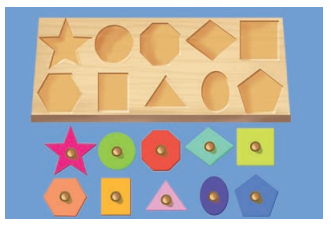Activity to be done by the students themselves